# Worksheet on Same Base and Same Parallels | Figures having Same Base and Same Parallels Worksheets

Students who are interested in learning the complete concept of geometric shapes having the same base and between the same parallels can refer to this page. Go through our Worksheet on Same Base and Same Parallels and prepare well for the competitive exams also. It includes the shortcuts, tricks, and detailed examples that are useful for the school students and one who is preparing for the jobs.

To get the complete knowledge of the same base and same parallels, learn and practice more example questions. Check the important points that say the relationship between the geometric shapes having the same base in the below sections.

## Relationship between Shapes Having Same Base and Same Parallels

The following are relations between the areas of few shapes lying on the same base and between the same parallels.

• If two parallelograms are on the same base and between the same parallels, those areas are equal.
• When a parallelogram and rectangle lies on the same base and between the same parallels, then the area of a parallelogram is equal to the area of the rectangle.
• If a parallelogram and triangle having the same base and are between the same parallels, then the area of a parallelogram is twice the area of the triangle.
• If a rectangle and a triangle lie on the same base and between the same parallels, then the area of the rectangle is equal to half of the area of the triangle.
• When Triangles on the Same Base and between the Same Parallel, then the area of triangles are equal.

1. In the parallelogram ABCD, E, F are any two points on sides AB and BC respectively. Show that area of ∆ ADF and the area of ∆ CDE are equal.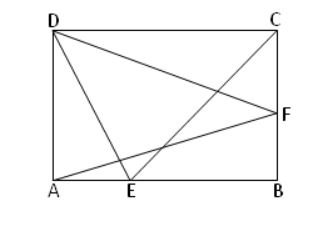Solution:

Draw a line from F perpendicular to AD and a line from E perpendicular to CD.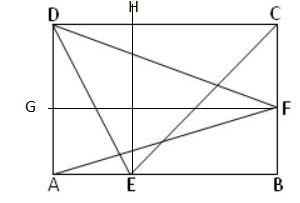Here, the triangle ADF and parallelogram ABCD are on the same base AD and between the same parallels CD and FG

We already know that Triangle and Parallelogram on the Same Base and between Same Parallels then the area of triangle is equal to the half of the area of the parallelogram.

So, the Area of △ ADF = 1/2 x Area of Parallelogram ABCD —- (i)

Here, CD is the common base for △ CDE, parallelogram ABCD, and the same parallels are BC and EH

In the same way, Area of △ CDE = 1/2 x Area of parallelogram ABCD —- (ii)

By observing the equation (i) and (ii) we can say that

Area of △ CDE = Area of △ ADF.

Hence shown.

2. Calculate the area of quadrilateral ABCD?Solution:

Hint: We can use Heron’s formula to find the area of triangle ABC and triangle ABD, then add those to get the quadrilateral area.

Semiperimeter of △ ABC s = (a + b + c)/ 2

s = 12 + 25 + 17)/2 = 54/2 = 27 units

Area of △ ABC = √[s (s – a) (s – b) (s – c)]

= √[27 (27 – 12) (27 – 25) (27 – 17)]

= √(27 x 15 x 2 x 10)

= √8100 = 90 square units.

Semiperimeter of △ ABD s = (12 + 20 + 28)/2 = 60/2 = 30 units

Area of △ ABD = √[s (s – a) (s – b) (s – c)]

= √[30 (30 – 12) (30 – 20) (30 – 28)]
= √(30 x 18 x 10 x 2)

= √10800 = 60√3 square units.

Area of quadrilateral ABCD = Area of △ ABD + Area of △ ABC

= 90 + 60√3 = 30(3 + 2√3) square units.

3. Parallelogram PQRS and rectangle PQUT are on the same base PQ and between the same parallels PQ and TR. Also, the area of the parallelogram is 108 cm² and the width of the rectangle is 6 cm. Find the length of the rectangle and its area.

Solution: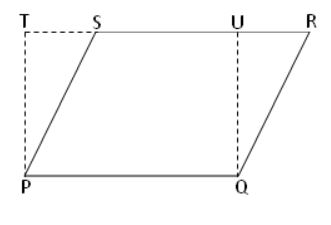Given that,

Area of the parallelogram = 108 cm²

Width of the rectangle = 6 cm

Area of the rectangle = length x width

We know that, if the parallelogram and rectangle lie on the same base and between the same parallels, then the area of the parallelogram is equal to the area of the rectangle.

So, Area of the parallelogram = Area of the rectangle

108 = length x width

108 = length x 6

length = 108/6

= 18 cm

Therefore, the rectangle length is 18 cm, and area is 108 cm².

4. Parallelogram PQRS and PQTU are on the same base PQ and between the same parallels PQ and UR. Area of parallelogram PQRS = 144 cm² and the altitude of the parallelogram PQTU = 16 cm. Find the length of the common side of two parallelograms.

Solution:Given that,

Area of the parallelogram PQRS = 144 cm²

The altitude of the parallelogram PQTU = 16 cm

We have two parallelograms PQRS and PQTU are on the same base PQ and between the same parallels PQ and UR.

So, the area of the parallelogram PQRS is equal to the area of parallelogram PQTU.

Area of the parallelogram PQRS = Area of the parallelogram PQTU

144 = base x altitude

144 = base x 16

base = 144/16

base = 9

Therefore, the length of the common side of two parallelograms is 9 cm.

5. In the adjoining figure, ∆ PQR is right-angled at Q in which QR = 12 cm and PQ = 4 cm. Find the area of ∆QSR; given that PS ∥ QR.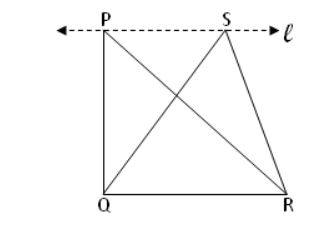Solution:

Given that,

QR = 12 cm, PQ = 4 cm

The triangles PQR and QRS lie on the same base QR and between the same parallels PS and QR.

Then the area of △ PQR = Area of △ QRS

1/2 x QR x PQ = 1/2 x QR x height

1/2 x 12 x 4 = 1/2 x 12 x height

24 = 6 x height

height of △ QRS = 24/6 = 4 cm

Then, area of ∆ QSR = 1/2 x 12 x 4

= 12 x 2 = 24 cm².

6. Parallelogram PQRS, ∆ PQS, and rectangle PQTU have the same base PQ. If the area of ∆ PSQ = 48 cm², then find the area of parallelogram PQRS and the area of rectangle PQTU.

Solution: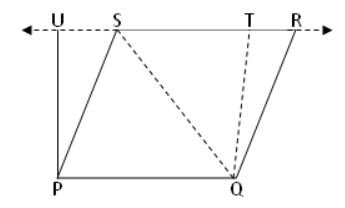Given that,

The area of ∆ PSQ = 48 cm²

Parallelogram PQRS and Triangle PQS are the same base PQ, and between the same parallels PQ, RS.

Then the area of parallelogram PQRS = Area of ∆ PSQ

Area of the parallelogram PQRS = 48 cm²

The parallelogram PQRS, rectangle PQTU having the same base and between the same parallels PQ and UT.

Then the area of parallelogram PQRS = Area of rectangle PQTU

Area of rectangle PQTU = 48 cm²

Therefore, the area of parallelogram PQRS is 48 cm² and the area of rectangle PQTU is 48 cm².

7. LM is median of ∆ JKL, JK = 15 cm, and the altitude of the ∆ JKL = 5 cm, find the area of ∆ JLM and area of ∆ LMK.

Solution: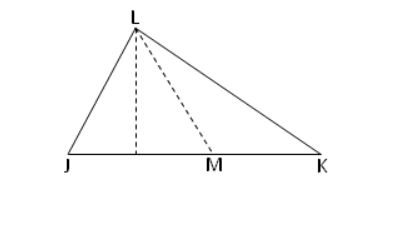Given that,

JK = 15 cm, altitude of the triangle JKL = 5 cm

Area of ∆ JKL = 1/2 x base x altitude of the triangle JKL

= 1/2 x 15 x 5 = 1/2 x 75

= 37.5 cm²

Triangle JKL and Triangle JLM lie on the same base JL and between the same parallel.

So, area of ∆ JKL = Area of ∆ JLM

Area of ∆ JLM = 37.5 cm²

Triangle JLM, triangle LMK is having the same base LM and between the same parallel

So, area of ∆ LMK = Area of ∆ JLM

Area of LMK = 37.5 cm²

Therefore, the area of ∆ JLM is 37.5 cm², and the area of ∆ LMK is 37.5 cm².

8. ∆ PQR is an isosceles triangle with ST//QR. The medians SR and QT intersect each other at O.

Prove that area of (1) ∆ QTS = ∆ RST

(2) ∆ QOS = ∆ ROT

(3) ∆ PQT = ∆ PRSSolution:

(1)

Given triangles QTS and RST are on the same base TS and between the same parallels.

The area of △ QTS = 1/2 x TS x OS

Area of △ RST = 1/2 x ST x OT

From the given figure, OT = OS

Area of △ RST = 1/2 x ST x OS

So, Area of △ RST = Area of △ QTS

Hence proved.

(2)

Triangle QOS and triangle ROT.

From the figure, we can say that OT = OS

Area of triangle QOS = 1/2 x OS x altitude of △ QOS

Area of triangle ROT = 1/2 x OT x altitude of △ ROT

= 1/2 x OS x altitude of △ ROT

the altitude of △ ROT = altitude of △ QOS

Therefore, Area of triangle QOS = Area of triangle ROT

Hence proved.

(3)

Area of triangle PQT = Area of triangle QST + Area of triangle PST

Area of triangle QST = 1/2 x ST x OS

Area of triangle PRS = Area of triangle RST + Area of triangle PST

Area of triangle RST = 1/2 x ST x OS

Area of triangle PRS = 1/2 x ST x OS + Area of trinagle PST

Area of triangle PQT = 1/2 x ST x OS + Area of triangle PST

Therefore, Area of triangle PRS = Area of triangle PQT

Hence proved.

9. Parallelogram PQRS, ∆PQS, and rectangle PQTU have the same base PQ. If the area of ∆PSQ = 56 cm², then find the area of parallelogram PQRS and the area of rectangle PQTU.

Solution:

Given that,The area of the triangle PSQ = 56 cm²

Parallelogram PQRS and Triangle PQS are the same base PQ, and between the same parallels PQ, RS.

Then the area of parallelogram PQRS = Area of ∆ PSQ

Area of the parallelogram PQRS = 56 cm²

The parallelogram PQRS, rectangle PQTU having the same base and between the same parallels PQ and UT.

Then the area of parallelogram PQRS = Area of rectangle PQTU

Area of rectangle PQTU = 56 cm²

Therefore, the area of parallelogram PQRS is 56 cm² and the area of rectangle PQTU is 56 cm².

10. Parallelogram PQRS and rectangle PQUT are on the same base PQ and between the same parallels PQ and TR. Also, the area of the parallelogram is 85 cm² and the width of the rectangle is 5 cm. Find the length of the rectangle and its area.

Solution:Given that,

Area of the parallelogram = 85 cm²

Width of the rectangle = 5 cm

Area of the rectangle = length x width

We know that, if the parallelogram and rectangle lie on the same base and between the same parallels, then the area of the parallelogram is equal to the area of the rectangle.

So, Area of the parallelogram = Area of the rectangle

85 = length x width

85 = length x 5

length = 85/5

= 17 cm

Therefore, the rectangle length is 17 cm, and the area is 85 cm².